• 逆时针旋转一角度旋转矩阵 cosθ -sinθ sinθ cosθ 这是坐标系顺时针 ,也就是坐标系中的点逆时针, 如果是坐标系逆时针 ,也就是坐标系中的点顺逆时针 只需将 θ 换成 -θ ,也就是 cosθ sinθ -sinθ cosθ ...
逆时针旋转某一角度的旋转矩阵
cosθ -sinθ
sinθ cosθ
这个是坐标系顺时针 ,也就是坐标系中的点逆时针,
如果是坐标系逆时针 ,也就是坐标系中的点顺逆时针
只需将 θ 换成 -θ ,也就是
cosθ sinθ
-sinθ cosθ


展开全文• ## 从基变换的角度理解旋转矩阵R

千次阅读 多人点赞 2020-12-23 16:49:05
从基变换的角度理解旋转矩阵R
在理解相机坐标系时，我们一定会接触相机的外参矩阵R，它将世界坐标系下的坐标转换到相机坐标系下：
$P_c=R*P_w+t$
这实际上是两个坐标系之间的变换，我们知道$R$矩阵是一个正交矩阵，所以它的3个行（列）向量是3维向量空间的一组标准正交基，而一组标准正交基可以作为一个坐标系的三个基向量。那么我们的$R$矩阵如何和两个坐标系的基向量联系起来呢？
我们先画出两个坐标系$X_wY_wZ_w$和$X_cY_cZ_c$：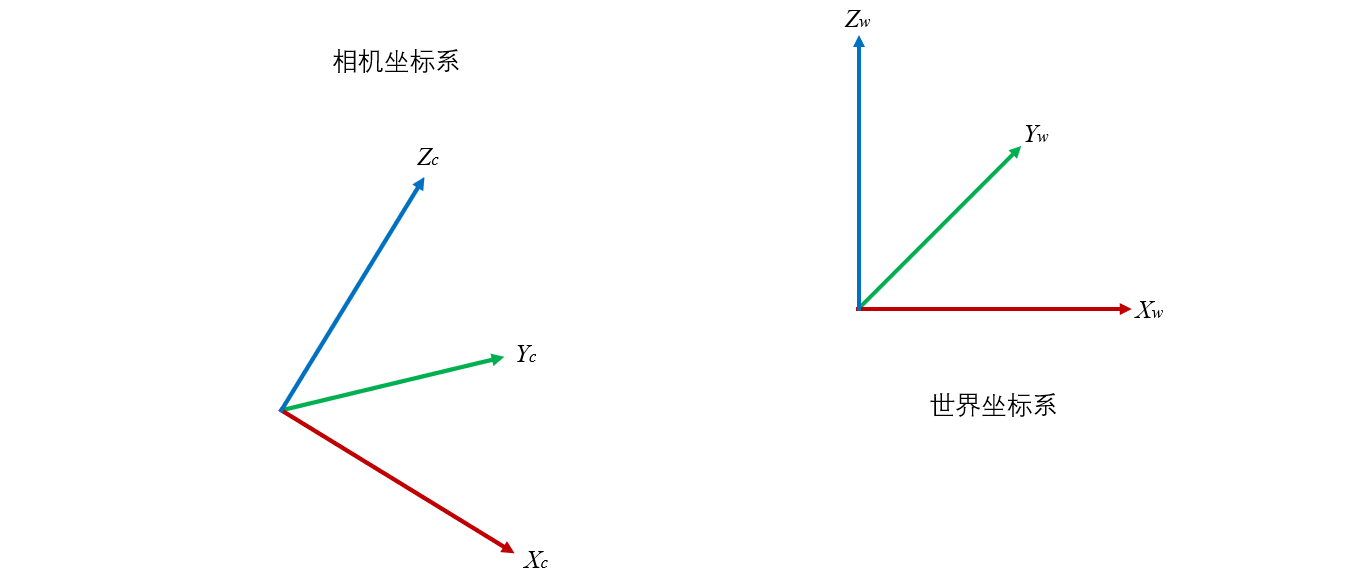我们要讨论的是如何把某一点 $P$ 在世界坐标系上的坐标转换成相机坐标系上的坐标。
暂且不考虑两个坐标系之间的平移，于是将相机坐标系的原点移动到世界坐标系的原点，像这样：我们可以标出两个坐标系的基向量组$e_w(\vec{e}_{wx},\vec{e}_{wy},\vec{e}_{wz})$和$e_c(\vec{e}_{cx},\vec{e}_{cy},\vec{e}_{cz})$。它们都在世界坐标系下。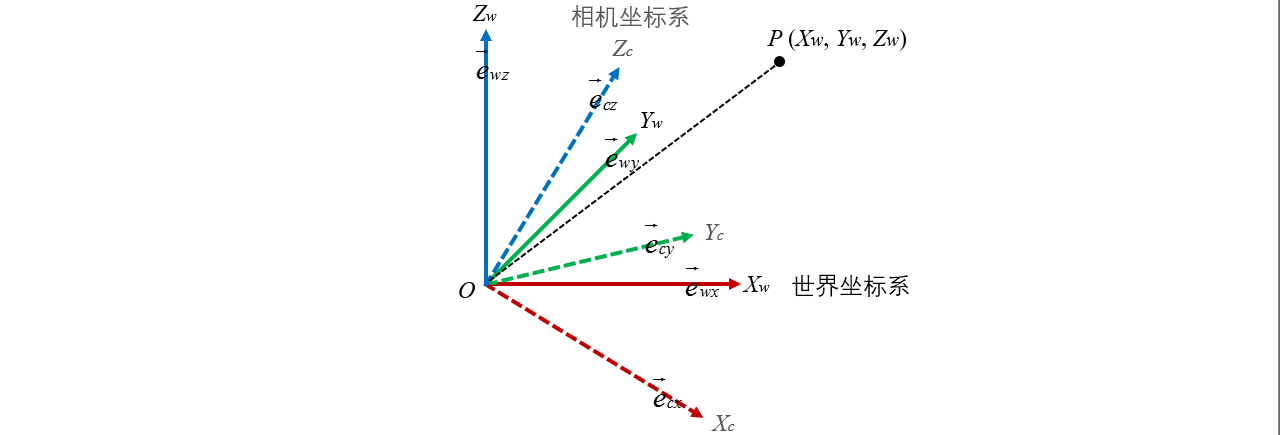接下来，再讨论如何把世界坐标系上的一点$P(X_w,Y_w,Z_w)$转换到相机坐标系下
$P(X_w,Y_w,Z_w)→P(X_c,Y_w,Z_w)$
在世界坐标系下，基向量组$e_w(\vec{e}_{wx},\vec{e}_{wy},\vec{e}_{wz})$为单位阵，也就是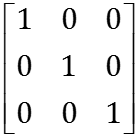其中$\vec{e}_{wx}=(1,0,0)^T$，$\vec{e}_{wy}=(0,1,0)^T$，$\vec{e}_{wz}=(0,0,1)^T$。
我们知道$P$在世界坐标系下的坐标实际上是以上三组基向量的线性组合，即$P_w=X_w*\vec{e}_{wx}+Y_w*\vec{e}_{wx}+Z_w*\vec{e}_{wx}$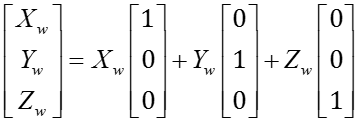这便是坐标的基向量表示法了。
那么我们把$P$点的坐标变换到基向量组$e_c(\vec{e}_{cx},\vec{e}_{cy},\vec{e}_{cz})$下便得到了相机坐标系下的变换。换句话说，我们要计算$P$点在基向量组$e_c(\vec{e}_{cx},\vec{e}_{cy},\vec{e}_{cz})$下的坐标$P_c=X_c*\vec{e}_{cx}+Y_c*\vec{e}_{cx}+Z_c*\vec{e}_{cx}$。
从旋转矩阵的角度来说，计算公式是：
$P_c=RP_w$
让我们先暂时忘掉$P$，我们想一想基向量组$e_c(\vec{e}_{cx},\vec{e}_{cy},\vec{e}_{cz})$通过$R$矩阵变换到相机坐标系下是什么样的呢？
答案显而易见，是单位阵$E$。
也就是说通过左乘旋转矩阵$R$，我们可以把基向量组$e_c(\vec{e}_{cx},\vec{e}_{cy},\vec{e}_{cz})$变成单位阵$E$，表达如下：
$R(\vec{e}_{cx},\vec{e}_{cy},\vec{e}_{cz})=E$
因此我们知道
$(\vec{e}_{cx},\vec{e}_{cy},\vec{e}_{cz})=R^{-1}=R^T$
这就是我们的旋转矩阵$R$在基变换角度下的理解，$R$的逆矩阵（或转置矩阵）的三个列向量，便是相机坐标系的三个基向量在世界坐标系下的坐标。


展开全文• ## 旋转变换（一）旋转矩阵

万次阅读 多人点赞 2017-03-27 17:15:37
本文主要介绍了计算机图形学中的旋转的概念和矩阵的描述方式，包括二维和三维旋转矩阵的推导过程
1. 简介

计算机图形学中的应用非常广泛的变换是一种称为仿射变换的特殊变换，在仿射变换中的基本变换包括平移、旋转、缩放、剪切这几种。本文以及接下来的几篇文章重点介绍一下关于旋转的变换，包括二维旋转变换、三维旋转变换以及它的一些表达方式（旋转矩阵、四元数、欧拉角等）。

2. 绕原点二维旋转

首先要明确旋转在二维中是绕着某一个点进行旋转，三维中是绕着某一个轴进行旋转。二维旋转中最简单的场景是绕着坐标原点进行的旋转，如下图所示：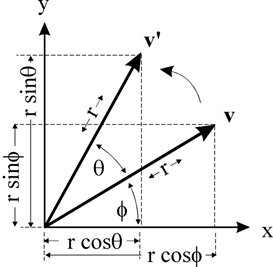如图所示点v 绕 原点旋转θ$\theta$ 角，得到点v’，假设 v点的坐标是(x, y) ，那么可以推导得到 v’点的坐标（x’, y’)(设原点到v的距离是r，原点到v点的向量与x轴的夹角是ϕ$\phi$ )
x=rcosϕy=rsinϕ$x = r cos\phi \quad y = r sin\phi$
x′=rcos(θ+ϕ)y′=rsin(θ+ϕ)$x' = r cos(\theta + \phi) \quad y' = rsin(\theta+\phi)$
通过三角函数展开得到
x′=rcosθcosϕ−rsinθsinϕ$x' = r cos\theta cos\phi - rsin\theta sin\phi$
y′=rsinθcosϕ+rcosθsinϕ$y' = rsin\theta cos\phi + r cos\theta sin\phi$
带入x和y表达式得到
x′=xcosθ−ysinθ$x' = xcos\theta - ysin\theta$
y′=xsinθ+ycosθ$y' = xsin\theta + ycos\theta$
写成矩阵的形式是：
[x′y′]=[cosθsinθ−sinθcosθ]∗[xy]
\begin{bmatrix}
x'\\
y'\\
\end{bmatrix}=        \begin{bmatrix}
cos\theta&-sin\theta\\
sin\theta&cos\theta\\
\end{bmatrix}*        \begin{bmatrix}
x\\
y\\
\end{bmatrix}

尽管图示中仅仅表示的是旋转一个锐角θ$\theta$的情形，但是我们推导中使用的是三角函数的基本定义来计算坐标的，因此当旋转的角度是任意角度（例如大于180度，导致v’点进入到第四象限）结论仍然是成立的。

3. 绕任意点的二维旋转

绕原点的旋转是二维旋转最基本的情况，当我们需要进行绕任意点旋转时，我们可以把这种情况转换到绕原点的旋转，思路如下：
1. 首先将旋转点移动到原点处
2. 执行如2所描述的绕原点的旋转
3. 再将旋转点移回到原来的位置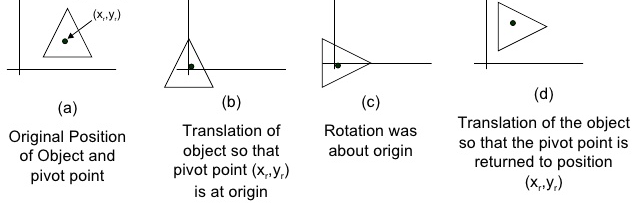也就是说在处理绕任意点旋转的情况下需要执行两次平移的操作。假设平移的矩阵是T(x,y)，也就是说我们需要得到的坐标 v’=T(x,y)*R*T(-x,-y)（我们使用的是列坐标描述点的坐标，因此是左乘，首先执行T(-x,-y)）

在计算机图形学中，为了统一将平移、旋转、缩放等用矩阵表示，需要引入齐次坐标。（假设使用2x2的矩阵，是没有办法描述平移操作的，只有引入3x3矩阵形式，才能统一描述二维中的平移、旋转、缩放操作。同理必须使用4x4的矩阵才能统一描述三维的变换）。

对于二维平移，如下图所示，P点经过x和y方向的平移到P’点，可以得到：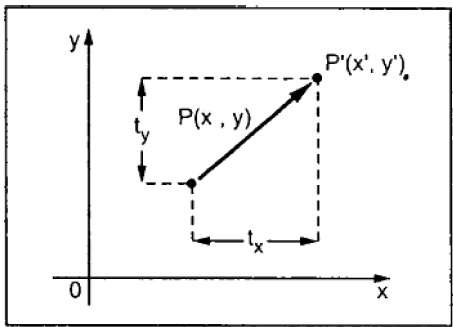x′=x+txy′=y+ty$x' = x + tx \quad y' = y+ty$
由于引入了齐次坐标，在描述二维坐标的时候，使用（x，y，w）的方式（一般w=1），于是可以写成下面矩阵的形式

⎡⎣⎢x′y′1⎤⎦⎥=⎡⎣⎢100010txty1⎤⎦⎥∗⎡⎣⎢xy1⎤⎦⎥
\begin{bmatrix}
x'\\
y'\\
1\\
\end{bmatrix}=        \begin{bmatrix}
1&0&tx\\
0&1& ty\\
0&0&1\\
\end{bmatrix}*        \begin{bmatrix}
x\\
y\\
1\\
\end{bmatrix}

按矩阵乘法展开，正好得到上面的表达式。也就是说平移矩阵是
⎡⎣⎢100010txty1⎤⎦⎥
\begin{bmatrix}
1&0&tx\\
0&1& ty\\
0&0&1\\
\end{bmatrix}

如果平移值是（-tx，-ty）那么很明显平移矩阵式
⎡⎣⎢100010−tx−ty1⎤⎦⎥
\begin{bmatrix}
1&0&-tx\\
0&1&-ty\\
0&0&1\\
\end{bmatrix}

我们可以把2中描述的旋转矩阵也扩展到3x3的方式，变为：
⎡⎣⎢x′y′1⎤⎦⎥=⎡⎣⎢cosθsinθ0−sinθcosθ0001⎤⎦⎥∗⎡⎣⎢xy1⎤⎦⎥
\begin{bmatrix}
x'\\
y'\\
1\\
\end{bmatrix}=
\begin{bmatrix}
cos\theta&-sin\theta&0\\
sin\theta&cos\theta&0\\
0&0&1\\
\end{bmatrix}*        \begin{bmatrix}
x\\
y\\
1\\
\end{bmatrix}

从平移和旋转的矩阵可以看出，3x3矩阵的前2x2部分是和旋转相关的，第三列与平移相关。有了上面的基础之后，我们很容易得出二维中绕任意点旋转的旋转矩阵了，只需要把三个矩阵乘起来即可：

M=⎡⎣⎢100010txty1⎤⎦⎥∗⎡⎣⎢cosθsinθ0−sinθcosθ0001⎤⎦⎥∗⎡⎣⎢100010−tx−ty1⎤⎦⎥=⎡⎣⎢cosθsinθ0−sinθcosθ0(1−cosθ)tx+ty∗sinθ(1−cosθ)ty−tx∗sinθ1⎤⎦⎥ M =               \begin{bmatrix}
1&0&tx\\
0&1& ty\\
0&0&1\\
\end{bmatrix}*      \begin{bmatrix}
cos\theta&-sin\theta&0\\
sin\theta&cos\theta&0\\
0&0&1\\
\end{bmatrix} *  \begin{bmatrix}
1&0&-tx\\
0&1&-ty\\
0&0&1\\
\end{bmatrix} = \begin{bmatrix}
cos\theta&-sin\theta&(1-cos\theta)tx + ty*sin\theta\\
sin\theta&cos\theta&(1-cos\theta)ty - tx*sin\theta\\
0&0&1\\
\end{bmatrix}

4. 三维基本旋转

我们可以把一个旋转转换为绕基本坐标轴的旋转，因此有必要讨论一下绕三个坐标值x、y、z的旋转。
本文在讨论过程中使用的是类似于OpenGL中定义的右手坐标系，同时旋转角度的正负也遵循右手坐标系的约定。如下图所示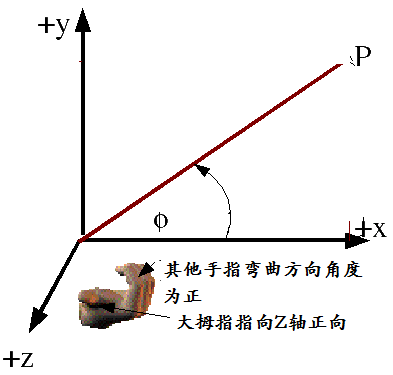4.1 绕X轴的旋转

在三维场景中，当一个点P(x,y,z)绕x轴旋转θ$\theta$角得到点P’(x’,y’,z’)。由于是绕x轴进行的旋转，因此x坐标保持不变，y和z组成的yoz（o是坐标原点）平面上进行的是一个二维的旋转，可以参考上图（y轴类似于二维旋转中的x轴，z轴类似于二维旋转中的y轴），于是有：
x′=x$\quad x' = x$
y′=ycosθ−zsinθ$\quad y' = ycos\theta - zsin\theta$
z′=ysinθ+zcosθ$\quad z' = ysin\theta + zcos\theta$
写成（4x4）矩阵的形式
⎡⎣⎢⎢⎢x′y′z′1⎤⎦⎥⎥⎥=⎡⎣⎢⎢⎢10000cosθsinθ00−sinθcosθ00001⎤⎦⎥⎥⎥∗⎡⎣⎢⎢⎢xyz1⎤⎦⎥⎥⎥        \begin{bmatrix}
x'\\
y'\\
z'\\
1\\
\end{bmatrix}= \begin{bmatrix}
1&0&0&0\\
0&cos\theta&-sin\theta&0\\
0&sin\theta&cos\theta&0\\
0&0&0&1\\
\end{bmatrix}* \begin{bmatrix}
x\\
y\\
z\\
1\\
\end{bmatrix}

4.2 绕Y轴旋转

绕Y轴的旋转和绕X轴的旋转类似，Y坐标保持不变，除Y轴之外，ZOX组成的平面进行一次二维的旋转（Z轴类似于二维旋转的X轴，X轴类似于二维旋转中的Y轴，注意这里是ZOX，而不是XOZ，观察上图中右手系的图片可以很容易了解到这一点），同样有：
x′=zsinθ+xcosθ$\quad x' = zsin\theta + xcos\theta$
y′=y$\quad y' = y$
z′=zcosθ−xsinθ$\quad z' = zcos\theta-xsin\theta$
写成（4x4）矩阵的形式
⎡⎣⎢⎢⎢x′y′z′1⎤⎦⎥⎥⎥=⎡⎣⎢⎢⎢cosθ0−sinθ00100sinθ0cosθ00001⎤⎦⎥⎥⎥∗⎡⎣⎢⎢⎢xyz1⎤⎦⎥⎥⎥        \begin{bmatrix}
x'\\
y'\\
z'\\
1\\
\end{bmatrix}= \begin{bmatrix}
cos\theta&0&sin\theta&0\\
0&1&0&0\\
-sin\theta&0&cos\theta&0\\
0&0&0&1\\
\end{bmatrix}* \begin{bmatrix}
x\\
y\\
z\\
1\\
\end{bmatrix}

4.3 绕Z轴旋转

与上面类似，绕Z轴旋转，Z坐标保持不变，xoy组成的平面内正好进行一次二维旋转（和上面讨论二维旋转的情况完全一样）
⎡⎣⎢⎢⎢x′y′z′1⎤⎦⎥⎥⎥=⎡⎣⎢⎢⎢cosθsinθ00−sinθcosθ0000100001⎤⎦⎥⎥⎥∗⎡⎣⎢⎢⎢xyz1⎤⎦⎥⎥⎥        \begin{bmatrix}
x'\\
y'\\
z'\\
1\\
\end{bmatrix}= \begin{bmatrix}
cos\theta&-sin\theta&0&0\\
sin\theta&cos\theta&0&0\\
0&0&1&0\\
0&0&0&1\\
\end{bmatrix}* \begin{bmatrix}
x\\
y\\
z\\
1\\
\end{bmatrix}

4.4 小结

上面描述了三维变换中绕单一轴旋转的矩阵表达形式，绕三个轴旋转的矩阵很类似，其中绕y轴旋转的矩阵与绕x和z轴旋转的矩阵略有点不同（主要是三个轴向顺序和书写矩阵的方式不一致导致的，绕三个不同坐标旋转轴以及其他二个坐标轴组成平面的顺序是： XYZ(绕x轴） YZX（绕y轴） ZXY（绕z轴），其中绕y轴旋转，其他两个轴是ZX，这和我们书写矩阵按 ⎡⎣⎢⎢⎢xyz1⎤⎦⎥⎥⎥\begin{bmatrix}
x\\
y\\
z\\
1\\
\end{bmatrix}
的方式不一致，而导致看起来绕Y轴旋转的矩阵似乎是和其他两个矩阵不一致。如果我们颠倒写法，将公式写成
⎡⎣⎢⎢⎢z′y′x′1⎤⎦⎥⎥⎥=⎡⎣⎢⎢⎢cosθ0sinθ00100−sinθ0cosθ00001⎤⎦⎥⎥⎥∗⎡⎣⎢⎢⎢zyx1⎤⎦⎥⎥⎥        \begin{bmatrix}
z'\\
y'\\
x'\\
1\\
\end{bmatrix}= \begin{bmatrix}
cos\theta&0&-sin\theta&0\\
0&1&0&0\\
sin\theta&0&cos\theta&0\\
0&0&0&1\\
\end{bmatrix}* \begin{bmatrix}
z\\
y\\
x\\
1\\
\end{bmatrix}

的方式，那么这三个旋转矩阵看起来在形式上就统一了，都是
[cosθsinθ−sinθcosθ]
\begin{bmatrix}
cos\theta&-sin\theta\\
sin\theta&cos\theta\\
\end{bmatrix}

这种表现形式了（左上角都是−sinθ$-sin\theta$）

5. 绕任意轴的三维旋转

绕任意轴的三维旋转可以使用类似于绕任意点的二维旋转一样，将旋转分解为一些列基本的旋转。绕任意轴旋转如下图所示：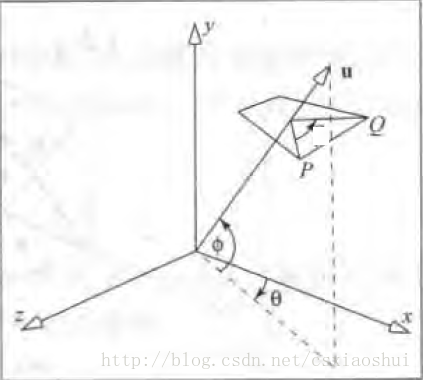P点绕向量u旋转θ$\theta$角，得到点Q，已知P点的坐标和向量u，如何求Q点的坐标。
我们可以把向量u进行一些旋转，让它与z轴重合，之后旋转P到Q就作了一次绕Z轴的三维基本旋转，之后我们再执行反向的旋转，将向量u变回到它原来的方向，也就是说需要进行的操作如下：
1. 将旋转轴u绕x轴旋转至xoz平面
2. 将旋转轴u绕y轴旋转至于z轴重合
3. 绕z轴旋转θ$\theta$角
4. 执行步骤2的逆过程
5. 执行步骤1的逆过程
原始的旋转轴u如下图所示：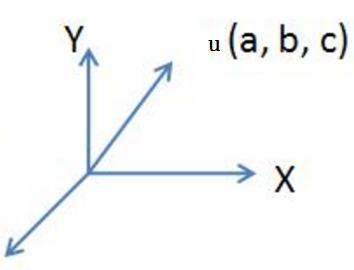第1、2、3步骤如下图所示：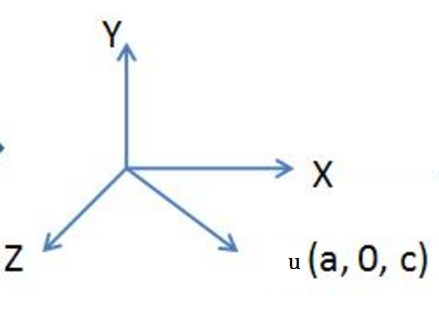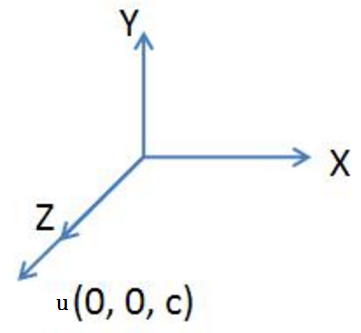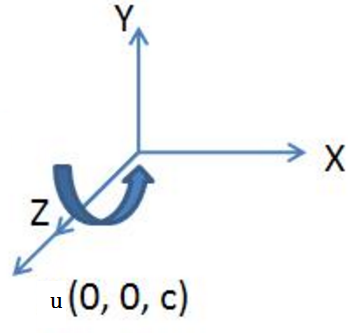步骤1将向量u旋转至xoz平面的操作是一个绕x轴的旋转操作，步骤2将向量u旋转到与z轴重合，第1、2步骤的示意图如下：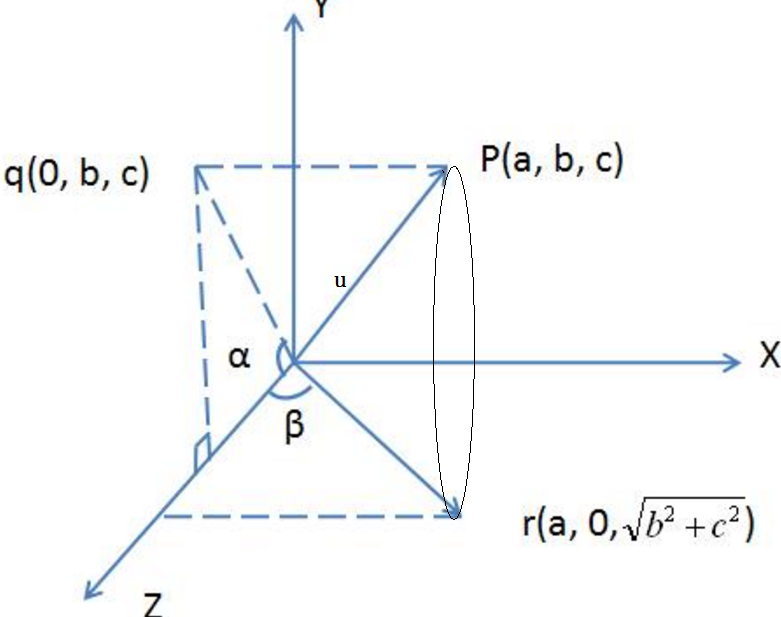作点P在yoz平面的投影点q，q的坐标是（0, b, c)，原点o与q点的连线oq和z轴的夹角就是u绕x轴旋转的角度。通过这次旋转使得u向量旋转到xoz平面（图中的or向量）【步骤1】
过r点作z轴的垂线，or与z轴的夹角为β$\beta$, 这个角度就是绕Y轴旋转的角度，通过这次旋转使得u向量旋转到与z轴重合【步骤2】

步骤1中绕x轴旋转的是一次基本的绕x轴的三维旋转，按照之前的讨论，旋转矩阵是：
⎡⎣⎢⎢⎢10000cosθsinθ00−sinθcosθ00001⎤⎦⎥⎥⎥     \begin{bmatrix}
1&0&0&0\\
0&cos\theta&-sin\theta&0\\
0&sin\theta&cos\theta&0\\
0&0&0&1\\
\end{bmatrix}

这里的θ$\theta$就是图中所示的α$\alpha$角 （注意α$\alpha$角度是绕x旋转的正的角度）
从图中我们还可以得到：
cosα=c(b2+c2)−−−−−−−√ cos\alpha = \frac{c}{\sqrt {(b^2+c^2)}}
sinα=b(b2+c2)−−−−−−−√ sin\alpha = \frac {b} {\sqrt {(b^2+c^2)}}
于是旋转矩阵(记作 Rx(α)$R_x(\alpha)$)为：
⎡⎣⎢⎢⎢⎢⎢⎢⎢⎢⎢⎢⎢10000c(b2+c2)−−−−−−−√b(b2+c2)−−−−−−−√00−b(b2+c2)−−−−−−−√c(b2+c2)−−−−−−−√00001⎤⎦⎥⎥⎥⎥⎥⎥⎥⎥⎥⎥⎥     \begin{bmatrix}
1&0&0&0\\
0&\frac{c}{\sqrt {(b^2+c^2)}}&-\frac {b} {\sqrt {(b^2+c^2)}}&0\\
0&\frac {b} {\sqrt {(b^2+c^2)}}&\frac{c}{\sqrt {(b^2+c^2)}}&0\\
0&0&0&1\\
\end{bmatrix}

在完成步骤1之后，向量u被变换到了r的位置，我们继续步骤2的操作，绕y轴旋转负的β$\beta$角（注意：这里的β$\beta$是负的），经过这次变换之后向量u与z轴完全重合，由于这一步也是执行的一次绕Y轴的基本旋转，旋转矩阵（记作 Ry(−β)$R_y(-\beta)$)为：
⎡⎣⎢⎢⎢cosθ0−sinθ00100sinθ0cosθ00001⎤⎦⎥⎥⎥   \begin{bmatrix}
cos\theta&0&sin\theta&0\\
0&1&0&0\\
-sin\theta&0&cos\theta&0\\
0&0&0&1\\
\end{bmatrix}

使用−β$-\beta$替换表达式中的θ$\theta$，此外根据图中描述，我们可以计算得到：
cosβ=(b2+c2)−−−−−−−√(a2+b2+c2)−−−−−−−−−−√ cos\beta = \frac{\sqrt {(b^2+c^2)}}{\sqrt {(a^2+b^2+c^2)}}
sinβ=a(a2+b2+c2)−−−−−−−−−−√ sin\beta = \frac {a} {\sqrt {(a^2+b^2+c^2)}}
带入上面的表达式，于是旋转矩阵(记作 Ry(−β)$R_y(-\beta)$)为：
⎡⎣⎢⎢⎢⎢⎢⎢⎢⎢⎢⎢⎢⎢⎢⎢(b2+c2)−−−−−−−√(a2+b2+c2)−−−−−−−−−−√0a(a2+b2+c2)−−−−−−−−−−√00100−a(a2+b2+c2)−−−−−−−−−−√0(b2+c2)−−−−−−−√(a2+b2+c2)−−−−−−−−−−√00001⎤⎦⎥⎥⎥⎥⎥⎥⎥⎥⎥⎥⎥⎥⎥⎥   \begin{bmatrix}
\frac{\sqrt {(b^2+c^2)}}{\sqrt {(a^2+b^2+c^2)}}&0&-\frac {a} {\sqrt {(a^2+b^2+c^2)}} &0\\
0&1&0&0\\
\frac {a} {\sqrt {(a^2+b^2+c^2)}} &0& \frac{\sqrt {(b^2+c^2)}}{\sqrt {(a^2+b^2+c^2)}}&0\\
0&0&0&1\\
\end{bmatrix}

在完成前面两个步骤之后，u方向和z轴完全重合，因此执行旋转θ$\theta$角，执行的是一次绕z轴的基本三维旋转（记作 R(θ)$R(\theta)$，根据之前的讨论，我们可以得到：
⎡⎣⎢⎢⎢cosθsinθ00−sinθcosθ0000100001⎤⎦⎥⎥⎥      \begin{bmatrix}
cos\theta&-sin\theta&0&0\\
sin\theta&cos\theta&0&0\\
0&0&1&0\\
0&0&0&1\\
\end{bmatrix}

最后两步骤是前面1和2的逆操作，也就是绕Y轴旋转β$\beta$ 和绕X轴旋转−α$-\alpha$，这两个矩阵分别记作 Ry(β)$R_y(\beta)$ 和 Rx(−α)$R_x(-\alpha)$，得到它们的方式很简单，只需要将上面步骤1和步骤2中的角度修改成相反数即可，也就是：

Ry(β)=⎡⎣⎢⎢⎢⎢⎢⎢⎢⎢⎢⎢⎢⎢⎢⎢(b2+c2)−−−−−−−√(a2+b2+c2)−−−−−−−−−−√0−a(a2+b2+c2)−−−−−−−−−−√00100a(a2+b2+c2)−−−−−−−−−−√0(b2+c2)−−−−−−−√(a2+b2+c2)−−−−−−−−−−√00001⎤⎦⎥⎥⎥⎥⎥⎥⎥⎥⎥⎥⎥⎥⎥⎥  R_y(\beta) =  \begin{bmatrix}
\frac{\sqrt {(b^2+c^2)}}{\sqrt {(a^2+b^2+c^2)}}&0&\frac {a} {\sqrt {(a^2+b^2+c^2)}} &0\\
0&1&0&0\\
- \frac {a} {\sqrt {(a^2+b^2+c^2)}} &0& \frac{\sqrt {(b^2+c^2)}}{\sqrt {(a^2+b^2+c^2)}}&0\\
0&0&0&1\\
\end{bmatrix}

Rx(−α)=⎡⎣⎢⎢⎢⎢⎢⎢⎢⎢⎢⎢⎢10000c(b2+c2)−−−−−−−√−b(b2+c2)−−−−−−−√00b(b2+c2)−−−−−−−√c(b2+c2)−−−−−−−√00001⎤⎦⎥⎥⎥⎥⎥⎥⎥⎥⎥⎥⎥   R_x(-\alpha)=  \begin{bmatrix}
1&0&0&0\\
0&\frac{c}{\sqrt {(b^2+c^2)}}&\frac {b} {\sqrt {(b^2+c^2)}}&0\\
0&-\frac {b} {\sqrt {(b^2+c^2)}}&\frac{c}{\sqrt {(b^2+c^2)}}&0\\
0&0&0&1\\
\end{bmatrix}

最终得到 绕任意轴u旋转的旋转矩阵是【因为使用的列向量，因此执行的是左乘（从右往左）】：

MR=Rx(−α)Ry(β)Rz(θ)Ry(−β)Rx(α)=M_R = R_x(-\alpha) R_y(\beta) R_z(\theta) R_y(-\beta) R_x(\alpha) =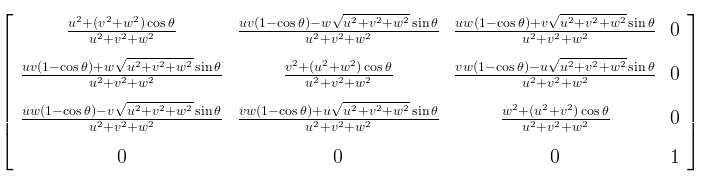(注：式中的(u,v,w)对应上文中向量(a,b,c)，公式我自己笔算过，为了减少编辑公式的时间（使用LaTex编辑太繁琐，因此找了一张公式的图片贴在此处)

如果向量是经过单位化的（单位向量），那么有a2+b2+c2=1$a^2+b^2+c^2 = 1$，可以简化上述的公式，得到：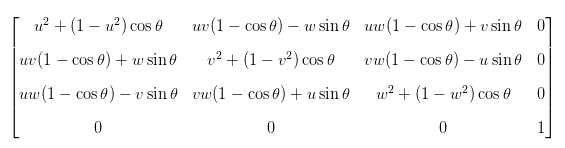参考文献：

Wiki Rotation (mathematics)
Euler’s rotation theorem
Maths - Rotation Matrices
绕任意轴旋转
Rotation About an Arbitrary Axis in 3 Dimensions
Rotation about an Arbitrary Axis (Line)


展开全文• 旋转角度 ...两个（不平行）的向量，决定一个平面， 而平面内任意向量，都可以通过旋转角度θ获得，所以，该平面的法向量一定是旋转轴（旋转轴不一定是该平面的法向量） 而法向量可以通过两向量的叉...


旋转角度
已知两向量a=(x1,y1,z1)和b=(x2,y2,z2),
两向量的旋转角度，可以通过点乘获得
a•b=|a|*|b|*cosθ
通过cosθ反转可求得旋转角度

旋转轴
两个（不平行）的向量，决定一个平面，
而平面内任意向量，都可以通过旋转角度θ获得，所以，该平面的法向量一定是旋转轴（旋转轴不一定是该平面的法向量）
而法向量可以通过两向量的叉乘得到，可参考
数学基础知识01

旋转矩阵
（旋转矩阵）是基于（旋转轴）和（旋转角度）推导出来的

具体推导公式，可参考前辈的以下网址
http://www.cnblogs.com/xpvincent/archive/2013/02/15/2912836.html

推导出来的（旋转矩阵）为公式说明
假设（旋转轴）的向量n=(nx, ny, nz);
I是单位矩阵，A是向量n的反对称矩阵，即反对称矩阵如何求，详情可看
数学基础知识01
而θ旋转角度

最后求出的旋转矩阵如下通过旋转矩阵可以求出（向量a）绕（旋转轴）旋转（角度θ）得到的（向量b）设3X3的（旋转矩阵）为R
v' = Rv

除此之外，在后面会遇到一种特殊的情况，（摄像机的移动）
已知（旋转轴向量n），已知（需要旋转的向量a），已知（需要旋转的向量a，逆时针旋转90度得到的向量b）
而且（向量n）是，（向量a）和（向量b），所在平面的法向量
通过旋转矩阵，我们可以得到化简整合有当（向量n）是法向量的时候，我们可以清楚地看见所以把vx2代入公式，有同理，把新得到的向量，逆时针旋转90度，可以获得，向量b旋转同样角度后，得到的新向量由x坐标变化，我们可以轻易获得向量变化之后的，y坐标 和 z坐标

转载于:https://www.cnblogs.com/keyncoin/p/5489598.html
展开全文• 在论文 Benchmarking 6DOF Outdoor Visual Localization in Changing Conditions 中看到的公式，根据旋转矩阵的估计值和真值计算角度误差。网上似乎相关资料也不多，记录一下。 Python代码 import numpy as np ...python
• %matlab测试代码 %这里随机生成两四元数 q1 和 q2 作为测试数据 ...%计算俩四元数之间的旋转角度差值（单位度数） 2*acos(abs(sum(q1.*q2)))*180/pi %将这两四元数转为旋转矩阵 r1 = quat2rot...
• ## 旋转向量-旋转矩阵

千次阅读 2015-07-22 17:32:24
今天接触到摄像机标定，其中用到旋转矩阵的知识，就具体恶补学习了一下，顺便做笔记。...如果已知这几个角度，就可以直接通过每一步的矩阵相乘得到整个旋转矩阵。 R=R(yaw)R(pitch)R(roll) R=R(yaw)R(pitcopencv
• 都知道旋转矩阵表达的是刚体（坐标系{B}）相对参考坐标系{A}的姿态信息，那如何利用已知的旋转矩阵，将{A}旋转一定角度变成与{B}一样的姿态呢？有几种方法：固定角旋转、欧拉角旋转、angle-axis表达法、Quaternion...欧拉角 齐次坐标
• 1，首先下载c++操作矩阵工具类Eigen，或者在这里下载。 2，下载后解压，把Eigen文件夹拷贝出来备用。 3，VS工程包含目录加上刚才文件夹的路径 4，加上头文件和命名空间 #include &lt;Eigen/Dense&gt; #...
• Problem Description ...每组输入先输入一个整数N（N &lt;= 50），代表矩阵的大小。 接下来的N行，每行有N个整数。代表矩阵A。 再接下来的N行N个整数代表矩阵B。 Output 如果矩阵A在旋转一定角度后能和矩阵B...
• 通常对于相机的位姿旋转都是采用旋转矩阵的方式来描述的，一个向量乘以旋转矩阵等价于向量以某种方式进行旋转。除了采用旋转矩阵描述外，还可以用旋转向量来描述旋转旋转向量的长度（模）表示绕轴逆时针旋转角度...
• 旋转矩阵 点、向量、坐标系 * 点——存在于三维空间之中，点和点组成向量，点本身由原点指向它的向量所描述 * 向量——带指向性的箭头，可以进行加法减法等运算，定义坐标系后，向量可以由R3R3 R^3 当中的...欧拉角
• 旋转矩阵1. 旋转矩阵简介 1. 旋转矩阵简介 旋转矩阵维基百科 在线性代数中，旋转矩阵是用于在欧几里得空间中进行旋转矩阵。例如，使用下面的约定， 矩阵：R=[cos(θ)−sin(θ)sin(θ)cos(θ)]R=\begin{bmatrix}...
• 一个平面坐标系逆时针旋转一个角度后得到另一个坐标系，则同一个点在这两个坐标系之间的几何关系如下： 由上图可得： 转化为矩阵表示为： 则反过来的关系如下： 由上面两个矩阵式可以看出两个转换的旋转矩阵是...机器学习
• 通过当前的旋转矩阵计算出当前这旋转矩阵对应的三轴的旋转角度： 参考连接：http://stackoverflow.com/questions/15022630/how-to-calculate-the-angle-from-roational-matrix 相关资料下载：...
• 任意旋转可用一个旋转轴和一个旋转角度来表示。 旋转向量，旋转向量的方向与旋转轴一致，长度为旋转角度。 在三维坐标下定义旋转轴为向量n(0,1,0)即旋转轴为y轴，旋转角度为k=30. 则旋转向量可用k*n表示。使用库...
• 定角（Fixed angles） 围绕固定的坐标系转动。固定坐标系的原点，坐标系再围绕已经固定的轴转动，全程原坐标系不动。...由角度旋转矩阵旋转矩阵角度 欧拉角（Euler angles） “自旋转..算法 线性代数
• 旋转向量为三维向量表示形式，用旋转向量表示旋转变换，可以将其方向为旋转轴方向，模为旋转角度。三维空间的旋转变换为绕任意轴r旋转角，可以分解为分别绕X,Y,Z轴旋转角，将绕坐标轴旋转表达为矩阵形式有： 图源...
• 任意输入两个9阶以下矩阵，要求判断第二个是否是第一个的旋转矩阵，如果是，输出旋转角度（0、90、180、270），如果不是，输出-1。 要求先输入矩阵阶数，然后输入两个矩阵，每行两个数之间可以用任意个空格分隔。行...
• 二维旋转矩阵推导： 点沿轴逆时针旋转度后变为点： 有， ...则旋转矩阵为 ...绕轴逆时针旋转角度旋转矩阵为： 绕轴逆时针旋转角度旋转矩阵为： 绕轴逆时针旋转角度旋转矩阵为： 则三维旋转矩阵为： ...
• 一个向量乘以旋转矩阵等价于向量以某种方式进行旋转。除了采用旋转矩阵描述外，还可以用旋转向量来描述旋转旋转向量的长度（模）表示绕轴逆时针旋转角度（弧度）。旋转向量与旋转矩阵可以通过罗德里格斯...
• OpenCV学习笔记（）——旋转向量与旋转矩阵相互转化   (2011-10-20 13:48:53) 转载▼ 标签：  宋体   旋转矩阵   向量   雅可比矩阵   罗德里格斯 分类： 软openCV...